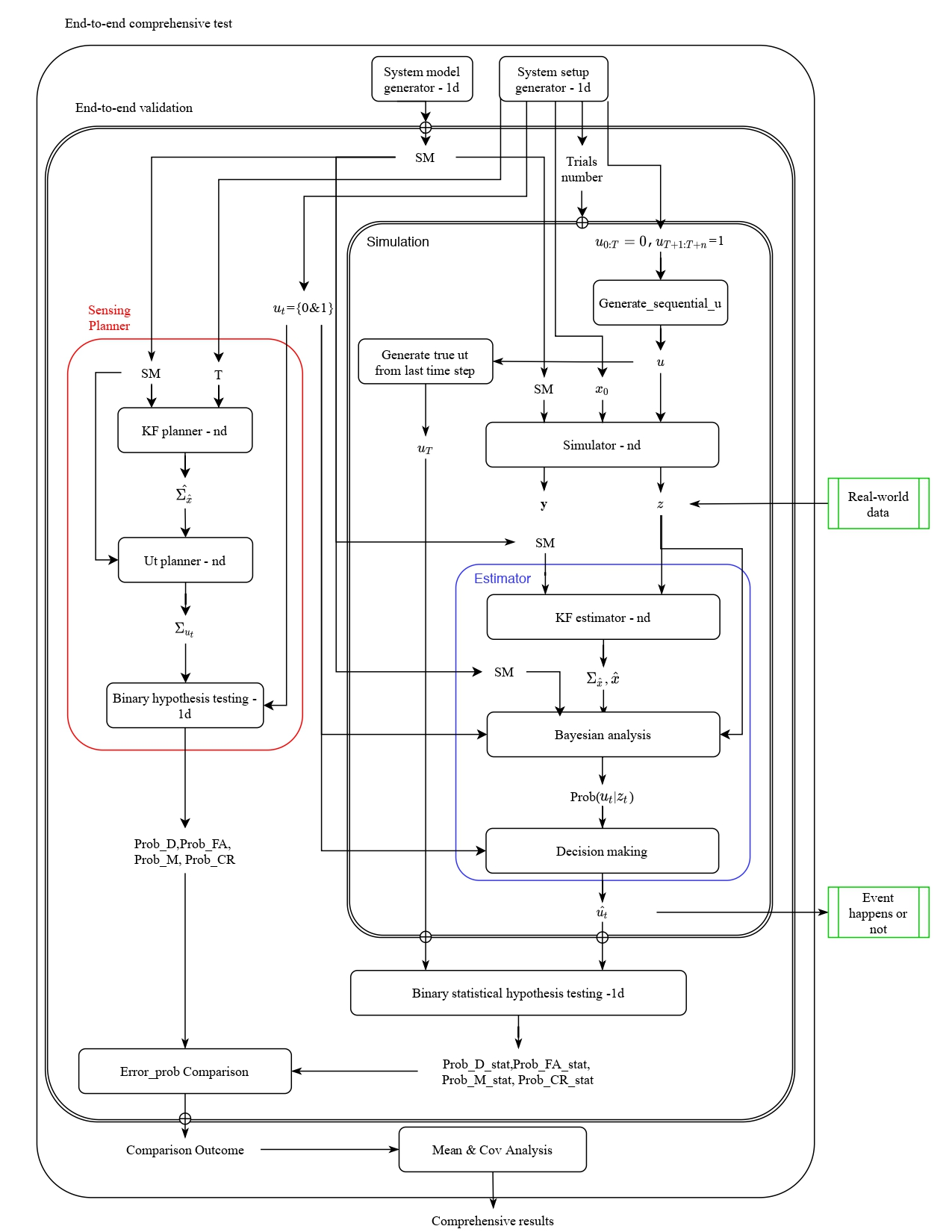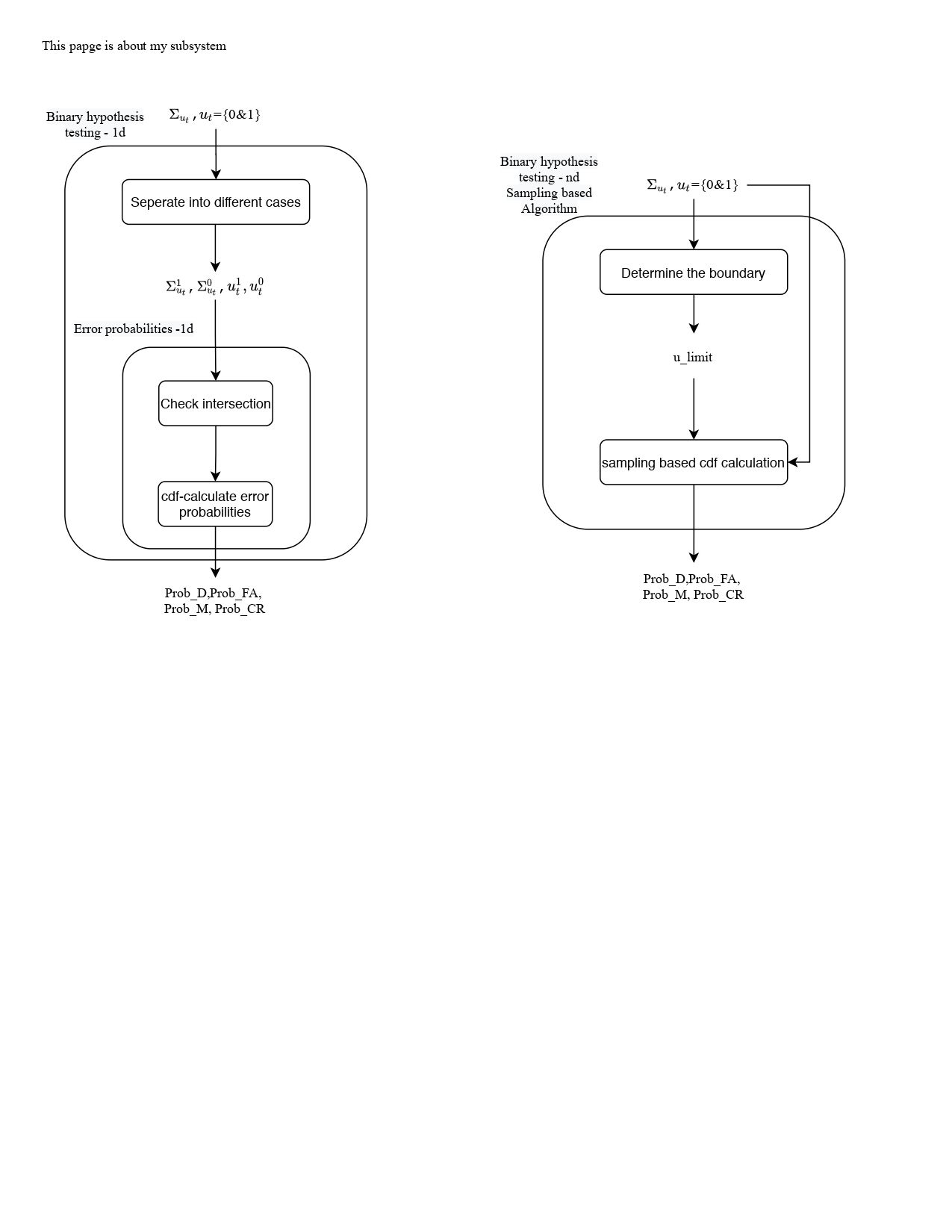04 Mar

#### Quick Notes of the Code of Darpa Create Project

This notes are quick notes after I have tested all Create code in practice. It contains the terms which easy to make people puzzled and somethings left to do. I marked this note in case to forget before I continue to finish the left parts.

### Important terms in code:

binary_1d: u (event) is binary values: 0/1, 1d means the u is 1 dimention (or x contains 1 sensor)

binary_nd: nd means u is n dimentions (or x contains n sensors)

multi_nd: u (event) has multi hypothesis and u is n dimentions (or x contains n sensors)

In code, multi-hypothesis of u event means there are multiple distributions list(miu_i, sigma_i), n-d means the dimention of u is n: u.ndim=(n,)

### Quick notes of Structure of the code:

• The algorithm can be divided into two parts. One is estimator branch based on statistical estimation of ut. Another is planner branch which based on theoratical estimation.

• The entrance of estimator is Simulations/Simulation.py; The entrance of planner is Sensor_planner/Sensor_planner.py.

• In this code, we generate numbers of System Model (SM) and each SM with numbers of trials. In each trials, we run the SM for T timestep and use the sequence of data of the T period as our test data.

• All system model (SM) parameters are set in E2Etest/System_setup_generator.py. [nHy = multiple hypothesis]

• All system models were generated in E2Etest/SM_generator_nd.py [ABHQR of SM)

Other code structure can be seen as the attached figures.

### Something hasn't been finished:

1. In etimator part (correponding to Simulations file):

Currently, it is only binary_1d esimator. To make it into binary_nd, we need to modify two functions: Estimator.Decision_making and Estimator.Bayesian_analysis; To turn it into multi_nd, we need to change SM_generator_1d and modify the above two functions.

2. In sensor planner part (corresponding to Sensor_planner file):

Currently, Sensor_planner.py lacks the last multi-hypothesis-test function.

3. End-to-end test:

Currently, we almost finished the code of estimator (namely simulation) and sensor planner. But we need to realize the end-to-end test to combine them into a whole. Currently, we almost finished the code of estimator (namely simulation) and sensor planner. But we need to realize the end-to-end test to combine them into a whole.

### Bugs need to discuss with Zhaoliang:

1. The function mul_Hyp_test_nd in Sensor_planner/mul_hypothesis_testing_nd.py may exist bugs. Because the output of Prob_errors is not a (4,) vector, it is the (nHy, nHy) matrix.

2. Error_prob_cal_oldV and Error_pro_cal in binary_HT_nd/Error_prob_cal.py

3. Complile error.（solved)

4. Question: how to plot in Spyder. It randomly came out the plot figures.